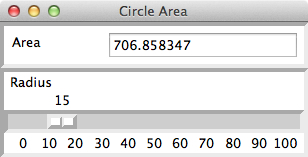Sliding Scales

A sliding scale (called a "scale" in Python and a "slider" in Java) allows the user to select a number by dragging a slider bar through an interval or range of values.  The interval can include integers or floating-point numbers.  The breezypythongui method addScale creates a new object of type Scale, adds it to the window, and returns the sliding scale object.  The row and column are, as usual, required attributes.  The next table shows the specific optional attributes that can be included when a sliding scale is created.

 Attribute Default value What it represents command lambda value: value The method triggered when a value changes during dragging.  value is the current value, as a string. from_ 0 The beginning value of the interval (displayed at the left or at the top). label "" The label displayed at the left or at the top of the sliding scale. length 100 (pixels) The size of the sliding scale along its orientation. orient HORIZONTAL The orientation of the sliding scale (can be set to VERTICAL). resolution 1 The interval between units of change detected during dragging. tickinterval 1 The interval between units that are displayed on the sliding scale. to 0 The ending value of the interval (displayed at the right or at the bottom).

The example program scaledemo1.py allows the user to view the area of a circle by dragging a slider bar through an interval of integers from 0 to 100.  Here is the interface for the program.As you can see, the sliding scale for the radius ranges from 0 to 100, with a tick interval of 10.  The current value, 15, is displayed above the position of the slider bar.  When the user moves the slider bar, this value and the area are updated automatically.  This scale has the default horizontal orientation.

Here is the code for the program.

from breezypythongui import EasyFrame

import math

class CircleArea(EasyFrame):

def __init__(self):

"""Sets up the window and widgets."""

EasyFrame.__init__(self, title = "Circle Area")

# Label and field for the area

row = 0, column = 0)

row = 0,

column = 1,

width = 20)

# Sliding scale for the radius

row = 1, column = 0,

columnspan = 2,

from_ = 0, to = 100,

length = 300,

tickinterval = 10,

command = self.computeArea)

# The event handler method for the sliding scale

"""Inputs the radius, computes the area,

and outputs the area."""

# radius is the current value of the scale, as a string.

area = float(radius) ** 2 * math.pi

self.areaField.setNumber(area)

# Instantiate and pop up the window.

CircleArea().mainloop()

Python’s Scale class also includes the methods get and set.  The method get takes no arguments and returns the sliding scale’s current value, as a number.  The method set expects a numeric argument and resets the sliding scale’s value to it.